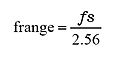FFT analyzer FAQWhat is the relationship between frequency range and sampling frequency?Though spectrum data for frequency bands up to 1/2 of a sampling frequency fs can be obtained in DFT (FFT) principles, actual FFT analyzers adopt an actual analysis range of a band smaller than 1/2 (1/2.56 of fs) in order to reduce aliasing error. This range is termed a frequency range (frange).

specifically, the relationship (1) results.(1)

Use of 2.56 derives from the fact that the FFT calculation point figure is a power of 2, and this decimal figure facilitates clipping of the frequency range. EU as the reference value in the same way as for the voltage value and is displayed as dBEU.

Ono Sokki FFT analyzers typically determine frequency range first, so conversely, the sampling frequency can be determined from Expression (1). For example, when the frequency range is 20 kHz, a 51.2 kHz sampling frequency results.

Revised:2010/12/20